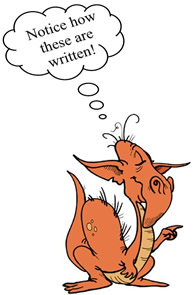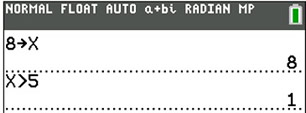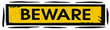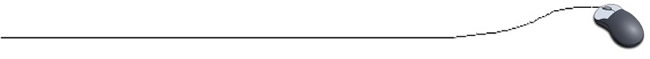Relational Operators

There are six relational (comparison) operators used for data comparisons in Java.Operator Description = = equal to ! = not equal to > greater than > = greater than or equal to < less than < = less than or equal to

Check out this numerical example:

 Assume these values are assigned: int a = 4; int b = 12; int c = 16; int d = 4; ... then the following are true: a = = d b < c c > a

 Notice that ONE equal sign is used to "assign" a value, but TWO equal signs are used to check to see if numerical values are equal to one another.

 Relations Operators in Java: Relational Operators on Graphing Calculator: Relational operators always yield a true or false result (not 1 or 0).   System.out.println(x > 5); The computer will display either the word TRUE or FALSE on the screen, depending upon the value stored in x.Certain calculators may display a 1 for TRUE and a 0 for FALSE.

Relational operators have a lower precedence than the arithmetic operators.
This means that an expression such as  x + 4 > = y - 5
is the same as (x + 4) > = (y - 5).Be careful with the use of == with Strings. Sometimes it looks as if = = compares String values. Using the = = operator, compares the memory addresses and not the actual string text. While use of = = may compile, it does not work reliably. Avoid!!! The most widely used method to compare String variables is the equals method. The equals method tests two String variables (or any two objects) to see if they have equal values. This equals method examines two strings, and if they contain the exact same string of characters, the strings are considered equal. This method is case sensitive. s1.equals(s2)       //checks for equality s1.equalsIgnoreCase(s2)  //checks for equality ignoring case String name = "tom"; String name2 = "joe"; if (name.equals(name2))       System.out.println("Yes"); else       System.out.prinln("No"); No will be printed on the screen.